CBSE Class 12 Sample Paper for 2023 Boards

Class 12
Solutions of Sample Papers and Past Year Papers - for Class 12 Boards

## In an elliptical sport field the authority wants to design a rectangular soccer field with the maximum possible area. The sport field is given by the graph of x 2 /a 2 +y 2 /b 2 =1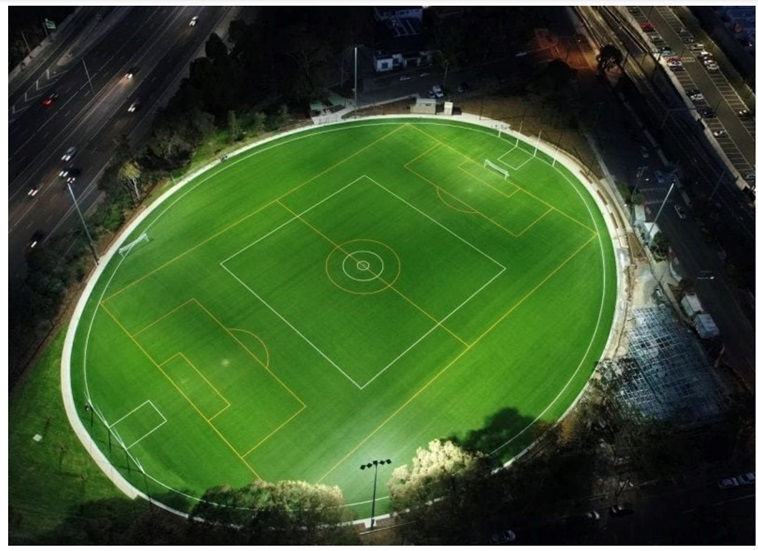## If the length and the breadth of the rectangular field be 2x and 2y respectively, then find the area function in terms of x.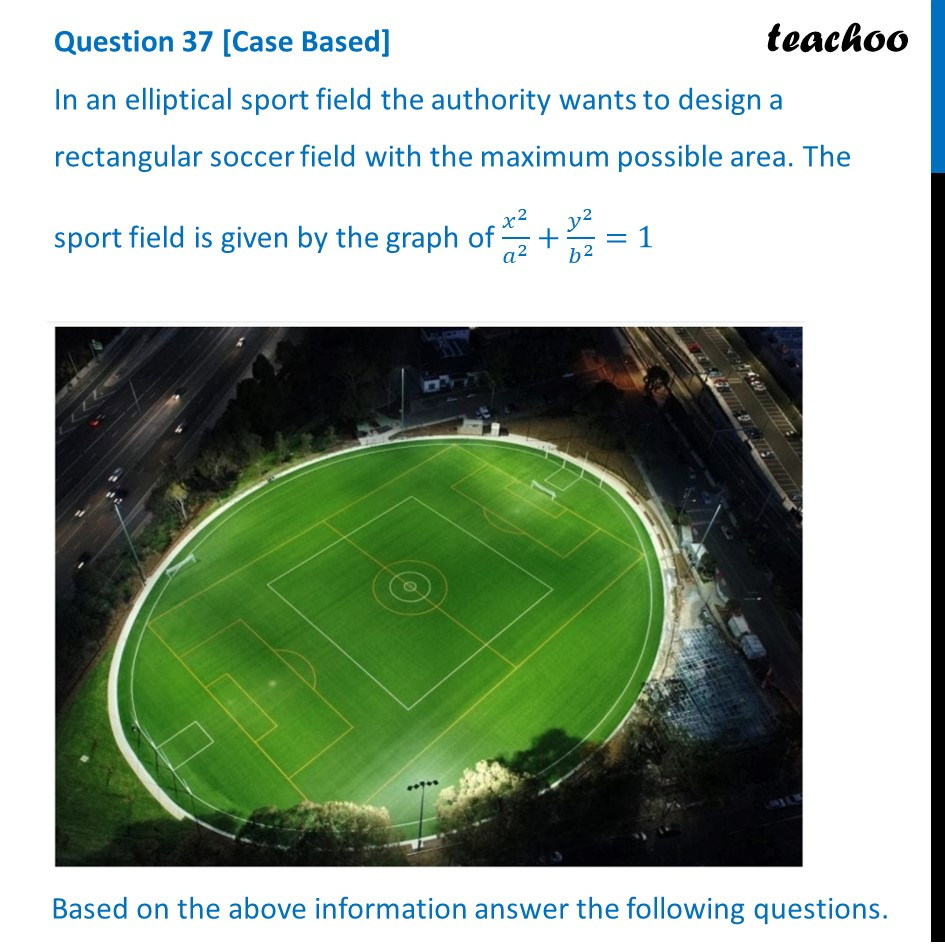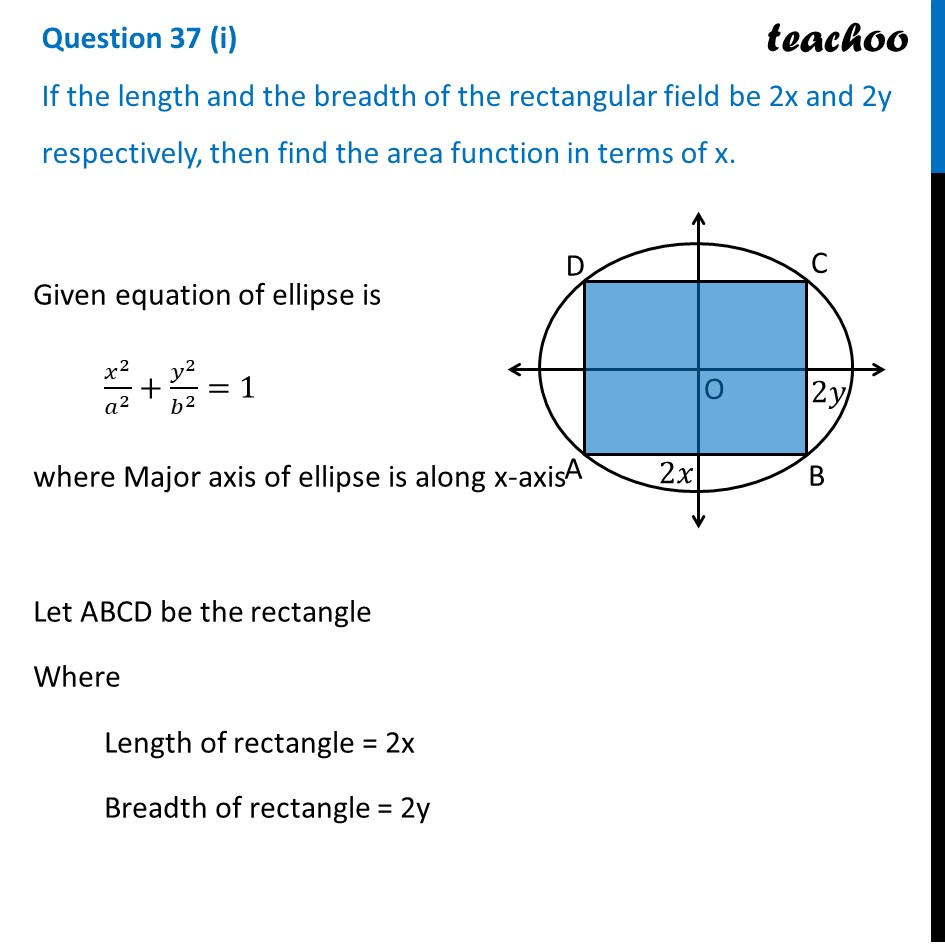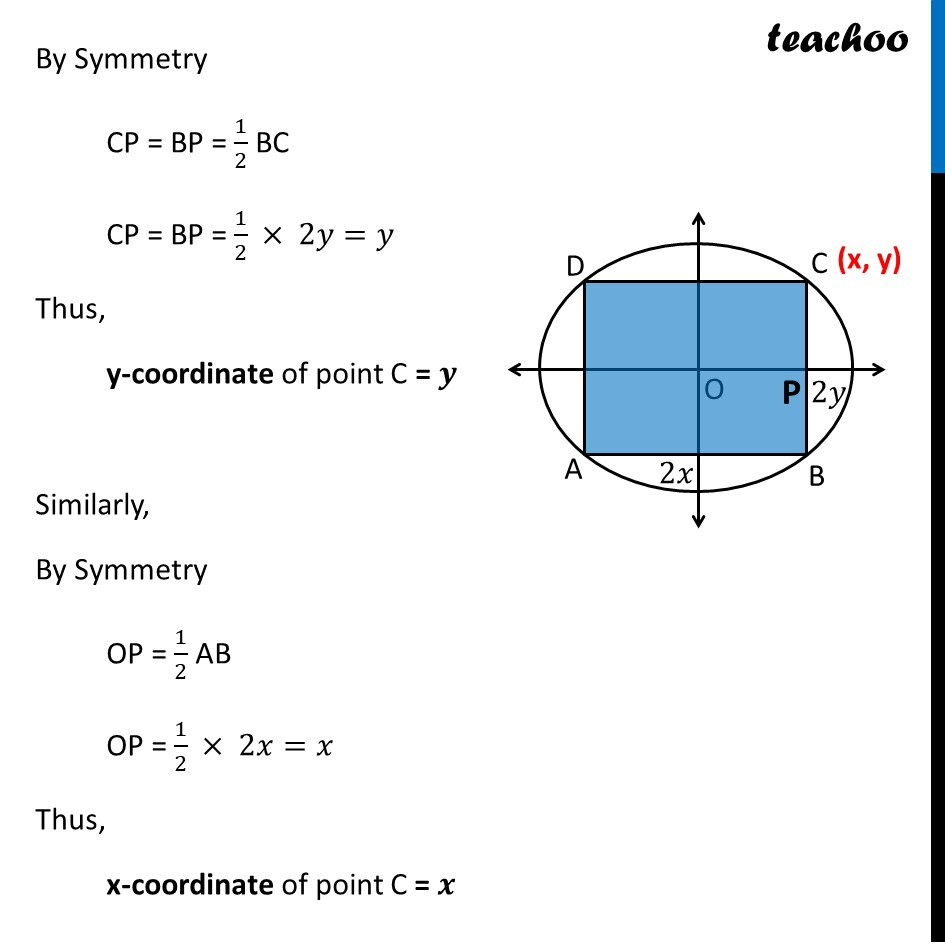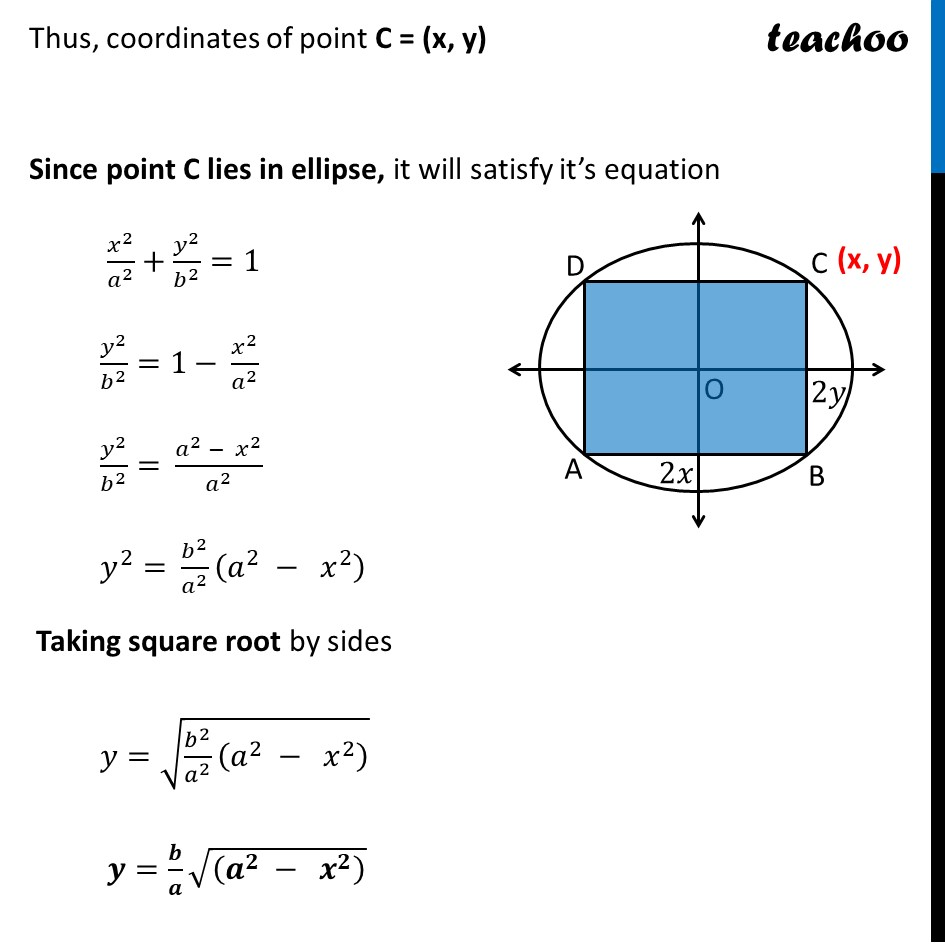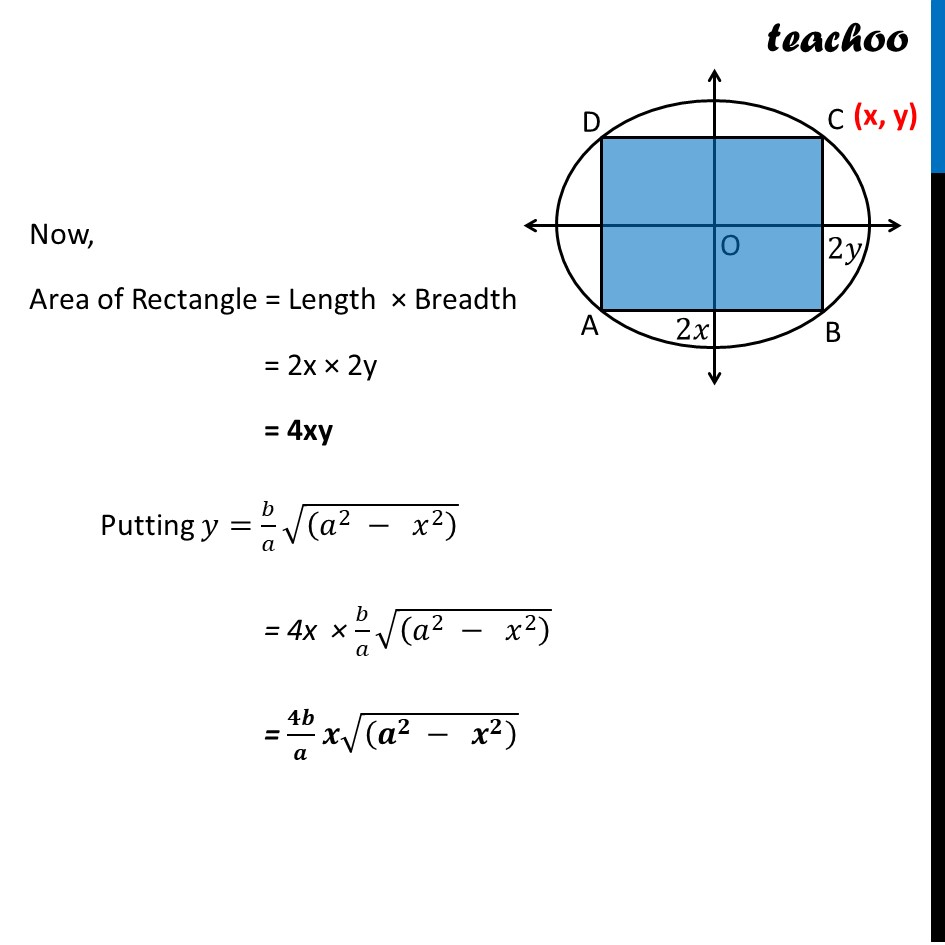Learn in your speed, with individual attention - Teachoo Maths 1-on-1 Class

### Transcript

Question 37 [Case Based] In an elliptical sport field the authority wants to design a rectangular soccer field with the maximum possible area. The sport field is given by the graph of 𝑥^2/𝑎^2 +𝑦^2/𝑏^2 =1Based on the above information answer the following questions. Question 37 (i) If the length and the breadth of the rectangular field be 2x and 2y respectively, then find the area function in terms of x.Given equation of ellipse is 𝑥^2/𝑎^2 +𝑦^2/𝑏^2 =1 where Major axis of ellipse is along x-axis Let ABCD be the rectangle Where Length of rectangle = 2x Breadth of rectangle = 2yBy Symmetry CP = BP = 1/2 BC CP = BP = 1/2 × 2𝑦=𝑦 Thus, y-coordinate of point C = 𝒚 Similarly, By Symmetry OP = 1/2 AB OP = 1/2 × 2𝑥=𝑥 Thus, x-coordinate of point C = 𝒙 Thus, coordinates of point C = (x, y) Since point C lies in ellipse, it will satisfy it’s equation 𝑥^2/𝑎^2 +𝑦^2/𝑏^2 =1 𝑦^2/𝑏^2 =1−" " 𝑥^2/𝑎^2 𝑦^2/𝑏^2 =" " (𝑎^2 − 𝑥^2)/𝑎^2 𝑦^2=" " 𝑏^2/𝑎^2 (𝑎^2 − 𝑥^2 ) Taking square root by sides 𝑦=" " √(𝑏^2/𝑎^2 (𝑎^2 − 𝑥^2 ) ) 𝒚=(" " 𝒃)/𝒂 √((𝒂^𝟐 − 𝒙^𝟐 ) ) Now, Area of Rectangle = Length × Breadth = 2x × 2y = 4xy Putting 𝑦=(" " 𝑏)/𝑎 √((𝑎^2 − 𝑥^2 ) ) = 4x × (" " 𝑏)/𝑎 √((𝑎^2 − 𝑥^2 ) ) = (" " 𝟒𝒃)/𝒂 𝒙√((𝒂^𝟐 − 𝒙^𝟐 ) )